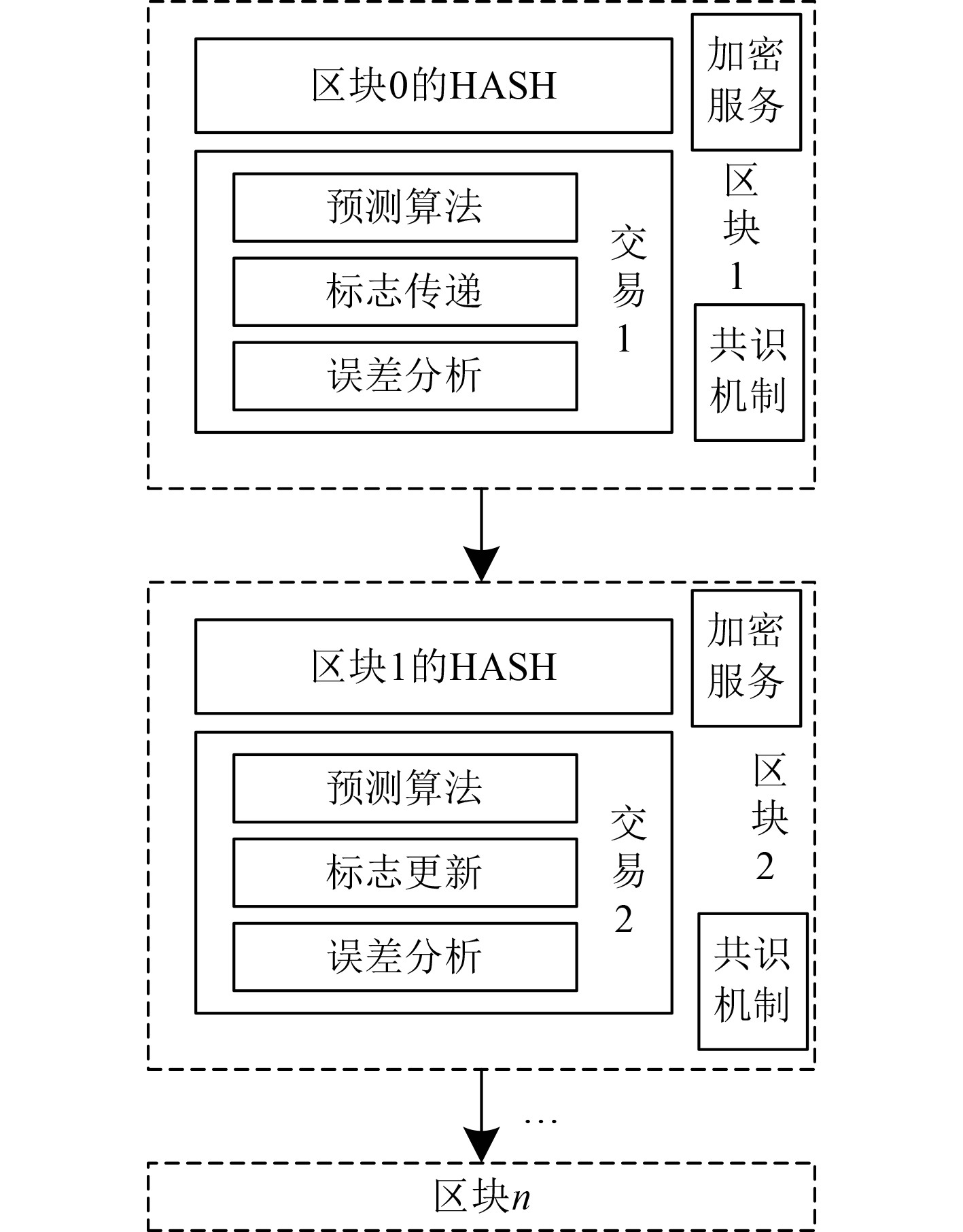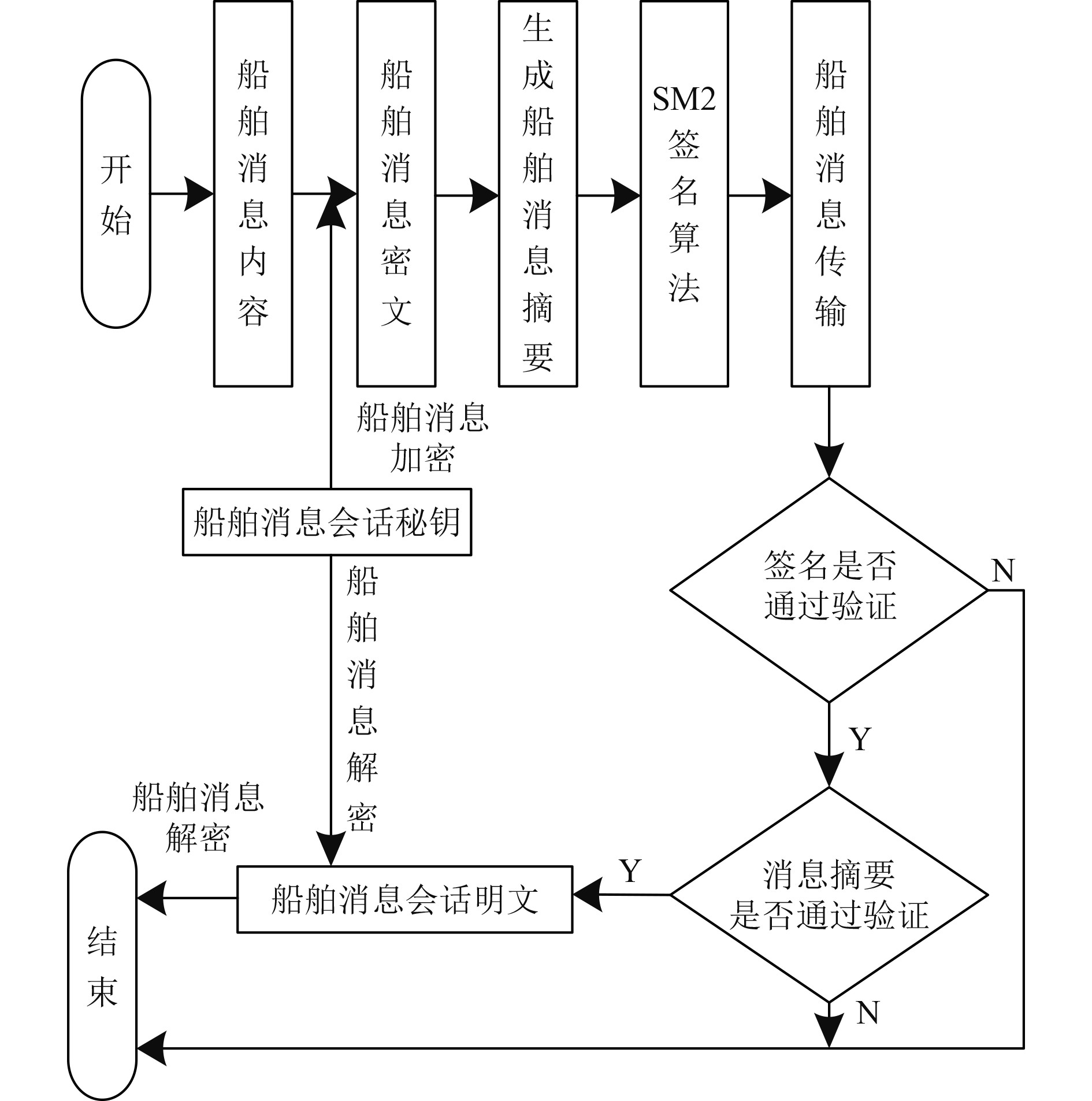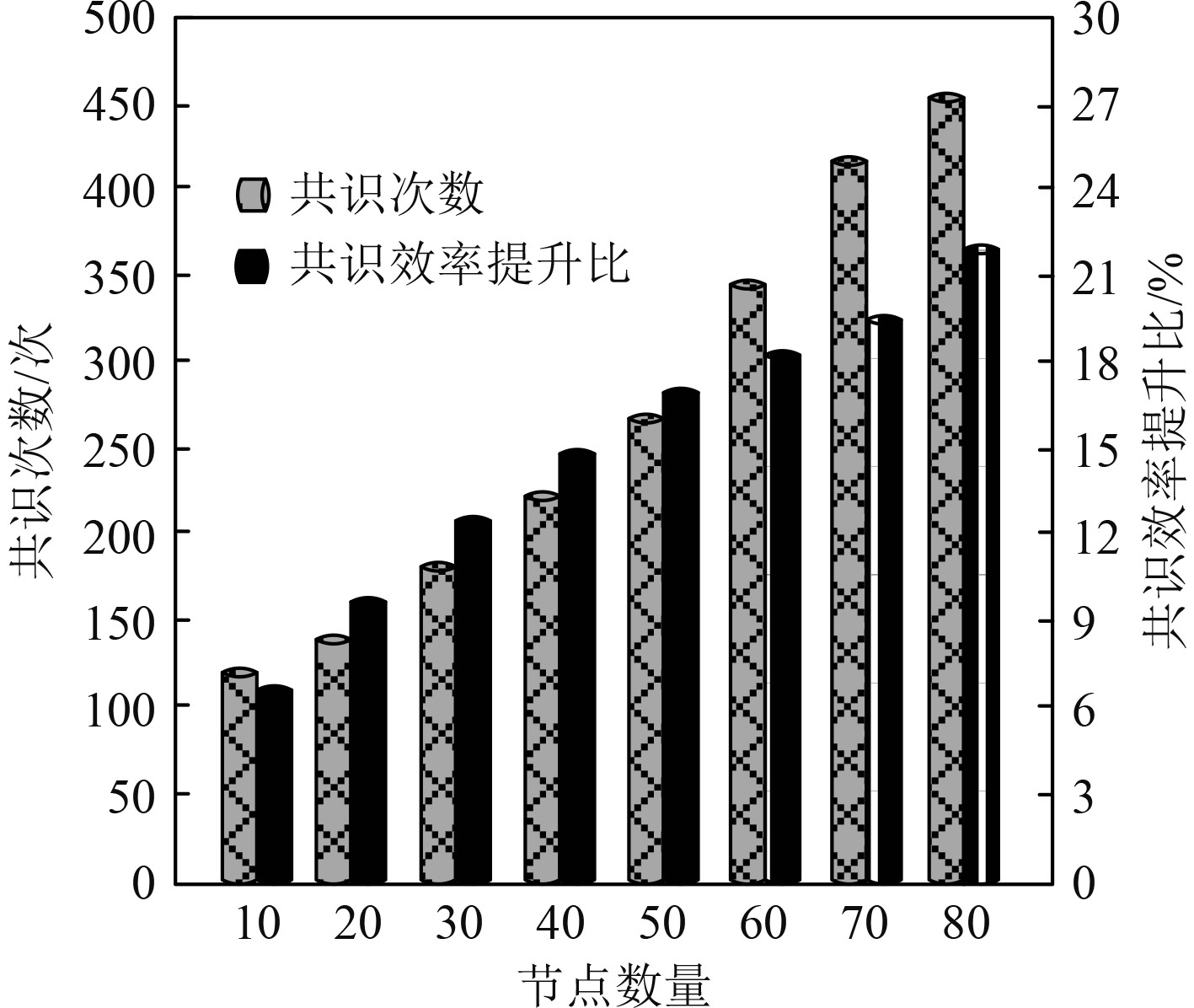﻿ 基于区块链技术的船舶信息安全预测
 舰船科学技术2022, Vol. 44Issue (10): 135-138    DOI: 10.3404/j.issn.1672-7649.2022.10.028PDF

Research on the prediction of ship information security based on blockchain technology
LIN De-li, DAI Lin-lin, YIN Cheng-bo
Qingdao Huanghai University, Qingdao 266000, China
Abstract: Research on the ship information security prediction method based on blockchain technology to improve the security of ship information transmission. The model chain is used to integrate blockchain technology and information security prediction algorithms to realize the secure transmission of ship information. The model chain selects the Boosting algorithm as the information security prediction algorithm to predict the decision-making power of the ship information transmission node. After determining the ship information transmission node with decision-making power, the blockchain technology adopts an improved consensus mechanism, and uses the blockchain encryption service provider to provide encryption services for ship information transmission. The blockchain encryption service provider selects the SM2 signature algorithm as the digital signature scheme for the blockchain encryption service to ensure the security of ship information transmission. The experimental results show that when the number of nodes in the ship communication system is 80, the consensus efficiency improvement ratio of blockchain technology is as high as 22%, which can realize the safe transmission of ship information.
Key words: blockchain technology     ship information     security prediction     boosting algorithm
0 引　言

1 区块链技术的船舶信息安全预测方法 1.1 基于区块链技术的船舶信息安全预测图 1 模型链结构图 Fig. 1 Model chain structure diagram
1.2 改进的区块链共识机制

1.3 区块链加密服务图 2 船舶信息加密解密流程图 Fig. 2 Flow chart of ship information encryption and decryption

1）形成秘钥

 ${Q_S} = d \times C，$ (1)

2）签名过程

 ${Z_A} = {H_{256}}\left( {{L_A}\left\| {a\left\| b \right.} \right.\left\| {{x_C}\left\| {{y_C}\left\| {{x_A}\left\| {{y_A}} \right.} \right.} \right.} \right.} \right)。$ (2)

 $e = \left( {{Z_A}\left\| M \right.} \right){H_{256}}，$ (3)

 ${X_1} = \left[ k \right] \times C，$ (4)

 $r = e\bmod n + {x_1}\bmod n ，$ (5)
 $s = {d^{ - 1}} \cdot \bmod n\left( {k - rd} \right)。$ (6)

3）签名验证过程

 $t = r' + s'\bmod n$ (7)

 ${X'_1} = \left[ {s' + t'} \right] + \left[ {C + {Q_S}} \right]$ (8)

1.4 Boosting算法的船舶信息传输节点决策权预测

Boosting算法确定船舶信息传输节点决策权的具体运行过程如下：

 ${\theta _m} = \sum\limits_{i = 1}^N {{w_m}\left( {{x_i},{y_i}} \right)}，$ (9)

 ${w_m} = \ln \frac{{1 - {\theta _m}}}{{2{\theta _m}}} ，$ (10)

 ${w_{m + 1}}\left( {{x_i},{y_i}} \right) = \frac{{{w_m}\left( {{x_i},{y_i}} \right){e^{ - {y_i}{f_m}\left( {{x_i}} \right)}}}}{{{W_m}}}，$ (11)

 $F\left( x \right) = \sum\limits_{m = 1}^M {{w_m}{f_m}} \left( x \right)。$ (12)
2 实验结果与分析表 1 传输节点决策权预测结果 Tab.1 Prediction results of decision rights of transmission nodes图 3 船舶信息加密结果 Fig. 3 Ship information encryption results表 2 本文方法应用性能 Tab.2 Application performance of the methods
3 结　语

  王玉英, 李岩, 李念峰. 基于区块链技术的网络文化信息安全传播机制与路径研究[J]. 情报科学, 2020, 38(10): 35-40.  洪耀球. 船舶油耗预测的机器学习算法比较研究[J]. 济南大学学报(自然科学版), 2021, 35(6): 546-551.  徐恪, 凌思通, 李琦, 等. 基于区块链的网络安全体系结构与关键技术研究进展[J]. 计算机学报, 2021, 44(1): 55-83.  高天航, 徐力, 靳廉洁, 等. 考虑航艏向与数据变化差异的船舶轨迹预测[J]. 交通运输系统工程与信息, 2021, 21(1): 90-94.  曾友渝, 谢强. 基于改进RNN和VAR的船舶设备故障预测方法[J]. 计算机科学, 2021, 48(6): 184-189.  李宗宣, 卜仁祥, 范艺. 结合速度和干扰观测的船舶路径跟踪模型预测控制[J]. 上海海事大学学报, 2021, 42(1): 19-25.  王少博, 张英俊, 胡鑫. 考虑船位预测不确定性的船舶碰撞危险度计算方法[J]. 中国舰船研究, 2021, 16(1): 114-120.  李孟特, 顾春华, 温蜜. 基于区块链的充电交易数据安全存储平台设计[J]. 计算机工程与应用, 2020, 56(21): 79-84.